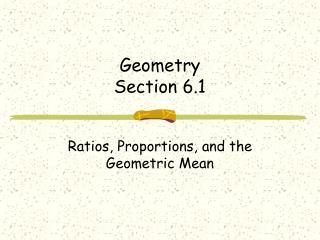DownloadDownload PresentationGeometry Section 6.1

# Geometry Section 6.1

Télécharger la présentation## Geometry Section 6.1

- - - - - - - - - - - - - - - - - - - - - - - - - - - E N D - - - - - - - - - - - - - - - - - - - - - - - - - - -
##### Presentation Transcript

1. GeometrySection 6.1 Ratios, Proportions, and the Geometric Mean

2. Ratio • The ratio of a to b is • Also written as a:b • Usually expressed in simplest form • 2 ratios that have the same simplified forms are called equivalent ratios

3. Examples • Simplify the ratio • 24 yards to 3 yards • 150 cm : 6 m • The perimeter of a room is 48 feet and the ratio of its length to its width is 7 : 5. find the length and width of the room.

4. Using extended ratios • A triangle’s angle measures are in the extended ratio of 1 : 3 : 5. find the measures of the angles.

5. Proportions An equation that states that the two ratios are equal. Cross product property. In a proportion the product of the extremes equals the product of the means.

6. Examples • Solve the Proportion

7. Example • As part of a science project, you need to estimate the number of blue spruce trees in a 50 acre forest. You count 36 trees in 3 acres and notice that the trees seem to be evenly distributed. Estimate the total number of blue spruce trees in the forest.

8. Geometric Mean • The geometric mean of two positive numbers a and b is the positive number x that satisfies so and

9. Examples • Find the geometric mean of the two numbers • 12 and 27 • 18 and 54 • 16 and 18

10. Assignment • Section 6.1 • Page 360 • Problems #4 – 44 even, 50